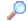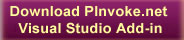SearchDirectory

Desktop Functions:

Smart Device Functions:GlossaryShow Recent ChangesSubscribe (RSS)Misc. Pages
SetCursor (user32)

Summary

#### C# Signature:

[DllImport("user32.dll")]
public static extern IntPtr SetCursor(IntPtr handle);

#### VB.Net Signature:

Declare Auto Function SetCursorPos Lib "user32.dll" (ByVal x As Integer, ByVal y As Integer) As Integer

<DllImport("user32.dll", SetLastError:=True)> _
Private Shared Function SetCursorPos(ByVal X As Integer, ByVal Y As Integer) As Boolean
End Function

None.

None.

#### Sample Code:

[DllImport("user32.dll")]
static extern bool ClientToScreen(IntPtr hwnd, ref Point lpPoint);

[DllImport("user32.dll")]
static extern bool SetCursorPos(int x, int y);

public void UnmanagedMoveCursorOverButton(Button button)
{
IntPtr handle = IntPtr.Zero;
Point point;
int x = 0;
int y = 0;
int width = 0;
int height = 0;
bool coordinatesFound = false;

if (button != null)
{
width = button.Size.Width;
height = button.Size.Height;
handle = button.Handle;
}

if ((width > 0) && (height > 0))
{
point = new Point();
x = (width / 2);
y = (height / 2);

coordinatesFound = ClientToScreen(handle, ref point);

if (coordinatesFound == true)
{
SetCursorPos(point.X + x, point.Y + y);
}
}
}

public void ManagedMoveCursorOverButton(Button button)
{
IntPtr handle = IntPtr.Zero;
Point point;
int x = 0;
int y = 0;
int width = 0;
int height = 0;
bool coordinatesFound = false;

if (button != null)
{
width = button.Size.Width;
height = button.Size.Height;
handle = button.Handle;
}

if ((width > 0) && (height > 0))
{
point = new Point();
x = (width / 2);
y = (height / 2);

coordinatesFound = ClientToScreen(handle, ref point);

if (coordinatesFound == true)
{
point.X += x;
point.Y += y;
System.Windows.Forms.Cursor.Position = point;
}
}
}

#### Alternative Managed API:

System.Windows.Forms.Cursor.Position

Documentation

Do you have...

• helpful tips or sample code to share for using this API in managed code?
• corrections to the existing content?
• variations of the signature you want to share?
• additional languages you want to include?

Select "Edit This Page" on the right hand toolbar and edit it! Or add new pages containing supporting types needed for this API (structures, delegates, and more).Access PInvoke.net directly from VS:Terms of UseEdit This PageFind ReferencesShow Printable VersionRevisions Show changes